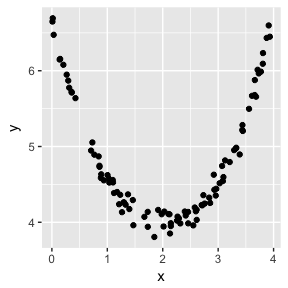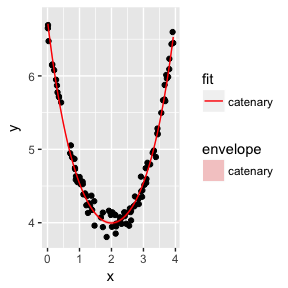# Simulate dataset

We simulate a dataset with x-points from a uniform (0,4) distribution, the y-points are from the function

$y_i = c_1 \cosh\left(\frac{x_i-c_2}{c_1}\right) + \lambda + \epsilon_i,$ where $$\epsilon_i \sim N(0,\sigma=0.1)$$.

library(catenary)
library(tidyverse)

sim_data <- data_frame(x = runif(100,0,4))
sim_data  <- sim_data %>%
mutate(y = f(x, c1 = 1, c2 = 2, lambda = 3))
sim_data  <- sim_data %>%
mutate(y = y + rnorm(100,0,0.1))

sim_data %>% ggplot(aes(x,y)) + geom_point()# Fitting a catenary to the data

We fit the catenary with the

sim_data_cat <- fittedCatenary(sim_data$x, sim_data$y)
##  "Fitted catenary"
plot(sim_data_cat,fit='cat', envelope='cat')
## Warning: Ignoring unknown aesthetics: yshow(sim_data_cat)
## $parameters ## value ## c1 1.000367 ## c2 1.997133 ## lambda 2.996144 ## ##$endpoints
##                x        y
## left  0.01005299 6.710548
## right 3.93234511 6.529990
##
## $length ## c1 ## 6.966456 ## ##$vertex
##     x.c2     y.c1
## 1.997133 3.996510
##
## \$ss
##     para catenary
## 1.109343 0.862067

## Get summary statistics of goodness of fit

Unfortunately R-square values are not appropriate for non-linear methods, which is how the catenary model is fitted to the data - see for example why-is-there-no-r-squared-for-nonlinear-regression. Instead I have added a new method gof() which will take a fitted catenary object and return the summary statistics like Akaike’s information criterion and Bayesian information criterion, which can be used to assess model fit.

gof(sim_data_cat)
##   model      sigma isConv       finTol   logLik       AIC       BIC
## 1   cat 0.09427242   TRUE 8.706064e-08 95.78577 -183.5715 -173.1509
## 2  para 0.10694169     NA           NA 83.17626 -158.3525 -147.9318
##   deviance df.residual r.squared adj.r.squared statistic      p.value df
## 1 0.862067          97        NA            NA        NA           NA NA
## 2 1.109343          97 0.9825454     0.9821855  2730.138 5.402863e-86  3